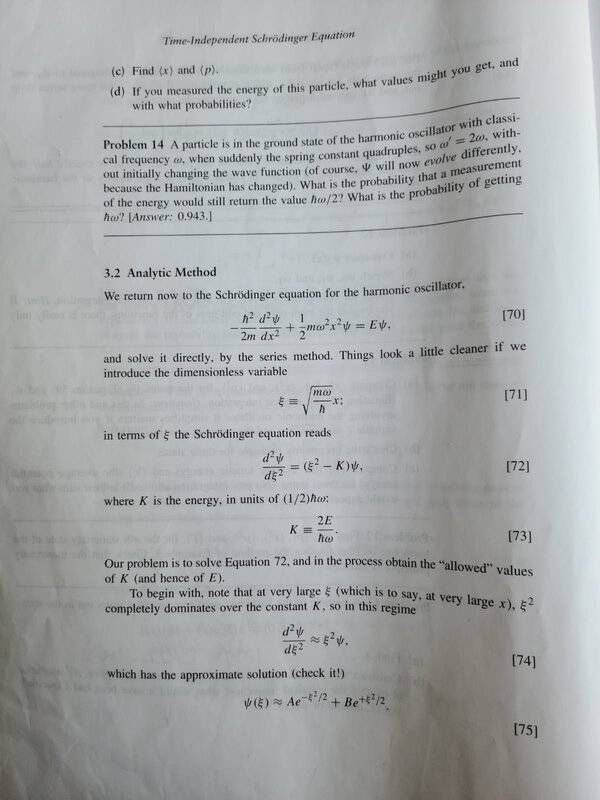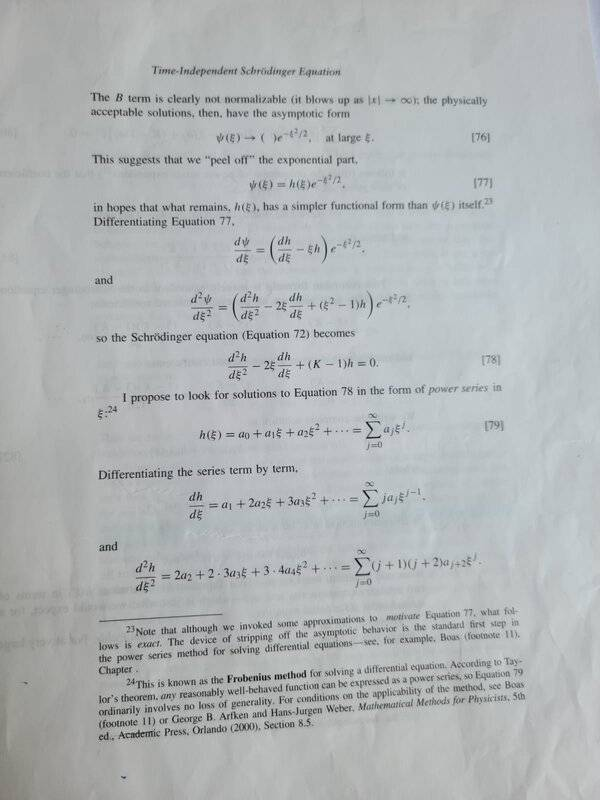# How to solve this 2nd order ODE?

• Tony Hau

#### Tony Hau

Homework Statement
Solve for ##\frac{d^{2}\psi}{d\xi^2} =\xi^2\psi##
Relevant Equations
For unequal roots 2nd order ODE, the solution is: ##y=c_{1}e^{-kx}+c_{2}e^{kx}##
This is a very simple question: I would like to solve for ##\psi## in this equation $$\frac{d^{2}\psi}{d\xi^2} =\xi^2\psi$$
I so apply ##y=c_{1}e^{-kx}+c_{2}e^{kx}## and ##\psi## should be equal to ##\psi=c_{1}e^{-\xi^2}+c_{2}e^{\xi^2}##, because ##(D^2-\xi^2)\psi=0##. However the answer is ##\psi(\xi) = c_{1}e^{-\frac{\xi^2}{2}}+c_{2}e^{\frac{\xi^2}{2}}##. Why is it so?

Your relevant equation is for constant coefficients. Your ODE does not have constant coefficients.

Try the substitution $\psi = e^{f(\xi)}$.

EDIT: Also, $(D - \xi)(D + \xi) \neq (D^2 - \xi^2)$ since multiplication by $\xi$ and differentiation wrt $\xi$ do not commute: By the product rule, $$D\xi = \xi D + 1.$$

Last edited:
•Tony Hau and Delta2
Additionally, $e^{\pm\xi^2/2}$ are not solutions, as can be determined by inspection: if $\psi = e^{\pm\xi^2/2}$ then \begin{align*} \psi' &= \pm \xi \psi \\ \psi'' &= \pm(\psi + \xi \psi') = (\xi^2 \pm 1)\psi \\ &\neq \xi^2 \psi. \end{align*} If you want to sove this ODE I suspect you will need to look for a power series. (My proposed substitution will lead to a first -order ODE in $f'$, but it has the drawback of being non-linear.)

•Tony Hau
Try tweaking pasmith's non solution. I.e. try e^(x^2/2) + f, and see what f needs to be to kill off the extra terms coming from the first part. Maybe the solution is not "elementary", since you need an antiderivative of e^(x^2/2).

•Tony Hau
Try tweaking pasmith's non solution. I.e. try e^(x^2/2) + f, and see what f needs to be to kill off the extra terms coming from the first part. Maybe the solution is not "elementary", since you need an antiderivative of e^(x^2/2).
I think it will turn out that ##f=e^{-\frac{x^2}{2}}##. In the OP he claims that the correct solution is ##\psi=c_1e^{\frac{x^2}{2}}+c_2e^{-\frac{x^2}{2}}## and I think that's correct.

I think I was wrong up to a constant, the solution seems to be $$\psi=c\left (e^{\frac{x^2}{2}}+e^{-\frac{x^2}{2}}\right )$$

•Tony Hau
Nope I was totally wrong this solution doesn't work. SORRY!

I think I was wrong up to a constant, the solution seems to be $$\psi=c\left (e^{\frac{x^2}{2}}+e^{-\frac{x^2}{2}}\right )$$
The solution is same as I stated. Actually this is from a proof on my textbook on quantum mechanics, where the proof is about solving the simple harmonic oscillator. ##\psi(x)## refers to the wave function; ##\xi## refers to the constant ##\sqrt{\frac{m\omega}{\hbar}}x##

The solution is same as I stated. Actually this is from a proof on my textbook on quantum mechanics, where the proof is about solving the simple harmonic oscillator. ##\psi(x)## refers to the wave function; ##\xi## refers to the constant ##\sqrt{\frac{m\omega}{\hbar}}x##
Can you post a screenshot from the book's page, cause the solution you state at the OP doesn't verify the differential equation.•Delta2
Can you post a screenshot from the book's page, cause the solution you state at the OP doesn't verify the differential equation.
By the way, it would be very nice if you could explain what asymptotic form is in the second picture. I don't understand the transition from ##\psi = Ae^{\frac{-\xi^2}{2}}+Be^{\frac{\xi^2}{2}}## to ##\psi = h(\xi)e^{\frac{-\xi^2}{2}}##

By the way, it would be very nice if you could explain what asymptotic form is in the second picture. I don't understand the transition from ##\psi = Ae^{\frac{-\xi^2}{2}}+Be^{\frac{\xi^2}{2}}## to ##\psi = h(\xi)e^{\frac{-\xi^2}{2}}##
TO be honest I don't understand that transition myself, however the book says that the solution you give at the OP is an approximate solution for large ##\xi##, something that you didn't tell us from start!

•Tony Hau
TO be honest I don't understand that transition myself, however the book says that the solution you give at the OP is an approximate solution for large ##\xi##, something that you didn't tell us from start!
I am sorry. I thought the approximation only refers to ##\frac{d^2\psi}{d\xi^2} \approx \xi^2\psi##; with regards to the approximation ##\frac{d^2\psi}{d\xi^2} \approx \xi^2\psi## , the following ##\psi = Ae^{-\frac{\xi^2}{2}}+ Be^{\frac{\xi^2}{2}}## is exact and is not an approximation.

I am sorry. I thought the approximation only refers to ##\frac{d^2\psi}{d\xi^2} \approx \xi^2\psi##; with regards to the approximation ##\frac{d^2\psi}{d\xi^2} \approx \xi^2\psi## , the following ##\psi = Ae^{-\frac{\xi^2}{2}}+ Be^{\frac{\xi^2}{2}}## is exact and is not an approximation.
No, ##\psi = Ae^{-\frac{\xi^2}{2}}+ Be^{\frac{\xi^2}{2}}## is not an exact solution of the ODE ##\frac{d^2\psi}{d\xi^2} = \xi^2\psi##. If you plug it in and calculate ##\frac{d^2\psi}{d\xi^2}## you ll find that it is equal to ##(\xi^2+1)\psi## as @pasmith first noted at post #3. But for large ##\xi## it is ##\xi^2+1\approx \xi^2## so it is indeed an approximate solution for large ##\xi## always.

•Tony Hau
This is a very simple question: I would like to solve for ##\psi## in this equation $$\frac{d^{2}\psi}{d\xi^2} =\xi^2\psi$$

This is a special case of Titchmarsh's Equation, the solution of which is in terms of special functions. In your case, the solution of ##y''-x^2y=0## is
$$y(x)=c_1 D[-1/2,\sqrt{2} x]+c_2 D[-1/2,i\sqrt{2}x]$$
where ##D[v,u]## is the parabolic cylinder function: Parabolic Cylinder function
Might be interesting to study how the approximate solution differs from this solution.

•Tony Hau and Delta2